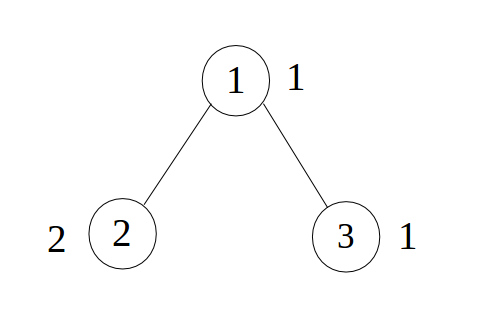Little Monk and Flip Operations
Tag(s):

## Algorithms, Bit manipulation, Depth-first search, Easy-Medium, Graph, Graph Theory

Problem
Editorial
Analytics

Given a tree with N nodes and one integer, a. Each node is numbered from 1 to N. Each node i has an integer, $A_i$, attached to it. You can perform only one type of operation, i.e.

1. Select a subtree of the given tree which includes the node a.

2. Select a non-negative integer k

3. Flip the $k^{th}$ least significant bit of the integers attached to each node in the selected subtree.

Note: A subtree of a tree T is a tree with both nodes and edges as subsets of nodes and edges of T.

Calculate the minimum number of operations that is required to make all the integers attached to the nodes of the given tree equal to 0.

Input format: :

First line contains two space separated integers, N and a $(1 \le N \le 10^5)$, $(1 \le a \le N)$. Next $N-1$ lines contains two space separated integers each, x and y $(1 \le x, y \le N)$, denoting that there is an edge between x and y. Next line contains N space separated integers, $A_i$ $(0 \le A_i \le 10^9)$, denoting the integers attached to the nodes.

Output format: :

Print the minimum number of operations that is required to make all the integers attached to the nodes of the given tree equal to 0.

SAMPLE INPUT
3 1
1 2
1 3
1 2 1

SAMPLE OUTPUT
3

ExplanationValues on each node in binary notation are:

$A_1 = 01$
$A_2 = 10$
$A_3 = 01$

In first operation, we will select node 1 and node 2 as subtree and $k = 2$. So, after flipping the $k^{th}$ least significant bit of the integers attached to each node in the selected subtree the new values on each node in binary notation will be:

$A_1 = 11$
$A_2 = 00$
$A_3 = 01$

In second operation, we will select node 1 as subtree and $k = 2$. So, after flipping the $k^{th}$ least significant bit of the integers attached to each node in the selected subtree the new values on each node in binary notation will be:

$A_1 = 01$
$A_2 = 00$
$A_3 = 01$

In third operation, we will select node 1 and node 3 as subtree and $k = 1$. So, after flipping the $k^{th}$ least significant bit of the integers attached to each node in the selected subtree the new values on each node in binary notation will be:

$A_1 = 00$
$A_2 = 00$
$A_3 = 00$

Time Limit: 1.0 sec(s) for each input file.
Memory Limit: 256 MB
Source Limit: 1024 KB
Marking Scheme: Marks are awarded when all the testcases pass.
Allowed Languages: Bash, C, C++, C++14, Clojure, C#, D, Erlang, F#, Go, Groovy, Haskell, Java, Java 8, JavaScript(Rhino), JavaScript(Node.js), Julia, Kotlin, Lisp, Lisp (SBCL), Lua, Objective-C, OCaml, Octave, Pascal, Perl, PHP, Python, Python 3, R(RScript), Racket, Ruby, Rust, Scala, Swift, Swift-4.1, TypeScript, Visual Basic

## CODE EDITOR

Initializing Code Editor...

## This Problem was Asked inChallenge Name

CodeMonk (Graph Theory Part I)

OTHER PROBLEMS OF THIS CHALLENGE
• Algorithms > Graphs
• Algorithms > Graphs
• Algorithms > Graphs
• Algorithms > Graphs